International
Tables for
Crystallography
Volume B
Reciprocal space
Edited by U. Shmueli

International Tables for Crystallography (2010). Vol. B, ch. 1.3, pp. 56-57   | 1 | 2 |

## Section 1.3.3.2.4. The Winograd algorithms

G. Bricognea

aGlobal Phasing Ltd, Sheraton House, Suites 14–16, Castle Park, Cambridge CB3 0AX, England, and LURE, Bâtiment 209D, Université Paris-Sud, 91405 Orsay, France

#### 1.3.3.2.4. The Winograd algorithms

| top | pdf |

The cyclic convolutions generated by Rader's multiplicative reindexing may be evaluated more economically than through DFTs if they are re-examined within a new algebraic setting, namely the theory of congruence classes of polynomials [see, for instance, Blahut (1985), Chapter 2; Schroeder (1986), Chapter 24].

The set, denoted, of polynomials in one variable with coefficients in a given fieldhas many of the formal properties of the setof rational integers: it is a ring with no zero divisors and has a Euclidean algorithm on which a theory of divisibility can be built.

Given a polynomial, then for everythere exist unique polynomialsandsuch thatand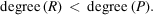is called the residue ofmodulo. Two polynomialsandhaving the same residue moduloare said to be congruent modulo, which is denoted byIf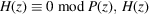is said to be divisible by. Ifonly has divisors of degree zero in, it is said to be irreducible over(this notion depends on). Irreducible polynomials play ina role analogous to that of prime numbers in, and any polynomial overhas an essentially unique factorization as a product of irreducible polynomials.

There exists a Chinese remainder theorem (CRT) for polynomials. Letbe factored into a product of pairwise coprime polynomials [i.e.andhave no common factor for]. Then the system of congruence equationshas a unique solutionmodulo. This solution may be constructed by a procedure similar to that used for integers. Let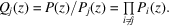Thenandare coprime, and the Euclidean algorithm may be used to obtain polynomialsandsuch thatWith, the polynomial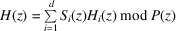is easily shown to be the desired solution.

As with integers, it can be shown that the 1:1 correspondence betweenandsends sums to sums and products to products, i.e. establishes a ring isomorphism:These results will now be applied to the efficient calculation of cyclic convolutions. Letandbe two vectors of length N, and letbe obtained by cyclic convolution of U and V:The very simple but crucial result is that this cyclic convolution may be carried out by polynomial multiplication modulo: ifthen the above relation is equivalent toNow the polynomialcan be factored over the field of rational numbers into irreducible factors called cyclotomic polynomials: if d is the number of divisors of N, including 1 and N, thenwhere the cyclotomicsare well known (Nussbaumer, 1981; Schroeder, 1986, Chapter 22). We may now invoke the CRT, and exploit the ring isomorphism it establishes to simplify the calculation offromandas follows:

 (i) compute the d residual polynomials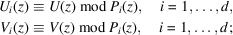(ii) compute the d polynomial products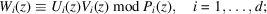(iii) use the CRT reconstruction formula just proved to recoverfrom the:When N is not too large, i.e. for short cyclic convolutions', theare very simple, with coefficients 0 or ±1, so that (i)only involves a small number of additions. Furthermore, special techniques have been developed to multiply general polynomials modulo cyclotomic polynomials, thus helping keep the number of multiplications in (ii)and (iii)to a minimum. As a result, cyclic convolutions can be calculated rapidly when N is sufficiently composite.

It will be recalled that Rader's multiplicative indexing often gives rise to cyclic convolutions of lengthfor p an odd prime. Sinceis highly composite for allother than 23 and 47, these cyclic convolutions can be performed more efficiently by the above procedure than by DFT.

These combined algorithms are due to Winograd (1977, 1978, 1980), and are known collectively as Winograd small FFT algorithms'. Winograd also showed that they can be thought of as bringing the DFT matrix F to the following normal form':where

 A is an integer matrix with entries 0,, defining the pre-additions', B is a diagonal matrix of multiplications, C is a matrix with entries 0,,, defining the `post-additions'.

The elements on the diagonal of B can be shown to be either real or pure imaginary, by the same argument as in Section 1.3.3.2.3.1.Matrices A and C may be rectangular rather than square, so that intermediate results may require extra storage space.

### References

Blahut, R. E. (1985). Fast Algorithms for Digital Signal Processing. Reading: Addison-Wesley.
Nussbaumer, H. J. (1981). Fast Fourier Transform and Convolution Algorithms. Berlin: Springer-Verlag.
Schroeder, M. R. (1986). Number Theory in Science and Communication, 2nd ed. Berlin: Springer-Verlag.
Winograd, S. (1977). Some bilinear forms whose multiplicative complexity depends on the field of constants. Math. Syst. Theor. 10, 169–180.
Winograd, S. (1978). On computing the discrete Fourier transform. Math. Comput. 32, 175–199.
Winograd, S. (1980). Arithmetic Complexity of Computations. CBMS-NST Regional Conf. Series Appl. Math, Publ. No. 33. Philadelphia: SIAM Publications.